52
[二年级数学]非常强大的小学100以内加减混合运算口算题大整理.doc
100-89+8=42-17+28=56-19+28= 36+4-24= 55+15-54=40+27-37= 54-34+3= 95-60+60= 45+30-21= 22+62-49=64+19-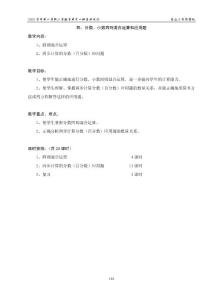42

2002学年第一学期小学数学第十一册备课笔记 爱山小学陈国权 118 四、分数、小数四则混合运算和应用题 教学内容： 1、四则混合运算 2、两步计算的分数（百分数）应用题 教学目标： 1、使学生能正确160

84+12=41-17= 97+45= 87-28= 38+23= 36+79= 9+69= 57-65= 11+82= 58+24= 39+41= 89-21= 41-33= 87+33= 16+3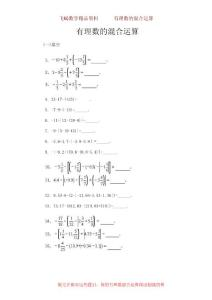36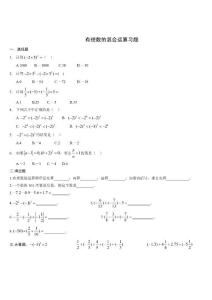53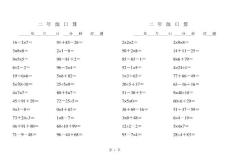22
【精品文献】小学二年级混合运算口算题.pdf
16－27＝91＋85－26＝ 21－0＝50＋28＝ 14＋11－25＝955＝ 90－81＋2＝ 85－83－1＝ 86＋79＝62－2＝ 96－24＝ 52－15＝ 4244＝19＋66＝ 56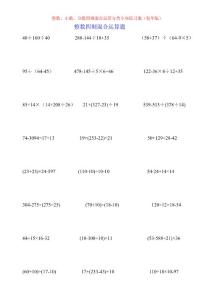60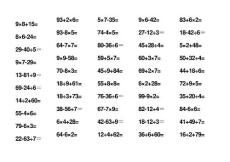20

98+15=86-24= 29-405＝ 97-29= 13-819＝ 69-246＝ 142+60= 55-46= 79-63= 22-637＝ 93+26= 93-85= 64-77= 99-58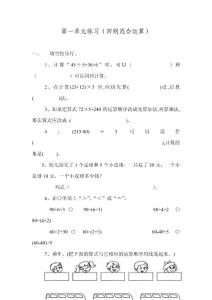6

、计算“455+366 ”时，可以（ ）可以同时计算。2、在计算(23+12) 3、如果把算式725+240 的运算顺序改成先算加法,再算乘法,那么算式应改成 5、幼儿园买了1个足球和5 个小皮球，一40

95%的用户最终下载了: